Next: The subs command Up: Preliminaries about Maple Previous: The diff (and Diff)

Subsections

# The int (and Int) command

## Symbolic integrals

The int command is used to compute both definite and indefinite integrals of Maple expressions. Its syntax is basically

>
int(f,x);


where f is an algebraic expression and x is the integration variable. For example, to compute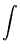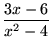dx ,

we execute the Maple command

>
int((3*x-6)/(x^2-4),x);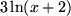Notice that Maple does not provide the constant of integration. You will occasionally have to take this into account and provide your own constant.

You must specify the variable of integration. In expressions involving other parameters, Maple assumes that you want the integral of the expression as the variable you specify changes and that all other parameters in the expression represent constants:

>
int(exp(a*x),x);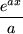To compute a definite integral, the range over which the integration variable moves must be specified:

>
int(x^2*exp(x),x=0..2);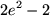The int command in Maple has a particular behavior in certain situations:

• If Maple cannot compute the integral in closed form, it will return it unevaluated:
>
int(ln(sin(sqrt(x^12-5*x^7+50*x+2))),x);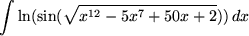• Sometimes the integral cannot be evaluated in closed form in terms of elementary functions, but the answer has a special name in mathematical circles due to its importance in applications. For example,
>
int(sin(2*x)/x,x);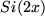Here Si is the special name of one of these functions which appear frequently in mathematical physics. To learn more about it, ask Maple for help on it with the command ?Si. This will bring up a window with information about the function Si. If Maple responds to an integral with one of these functions, it is quite likely that the integral cannot be evaluated in terms of elementary functions.

• Sometimes, a result may be expressed in terms of the roots of a polynomial which does not factor over the rationals. For example,

>
int(1/(x^8 +1),x);In this answer, the sum is taken over all roots R of the polynomial 1 + 16777216z8, and the summand is R log(x+8R).

As with diff, there is an inert form Int of the integral command which can be used in combination with int to produce easily readable worksheets:

>
Int(ln(1+3*x), x=1..4);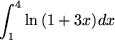>
Int(ln(1+3*x), x=1..4)=int(ln(1+3*x),x=1..4);The inert form Int is also very useful in many situations when you wish to delay the evaluation of an integral, as we shall see below.

## Numerical integration

With the evalf command, you can force Maple to apply a numerical approximation technique for definite integration:
>
evalf(Int(sqrt(1+x^10),x=0..1));Notice that the we are using the inert form of the integration command, Int. This prevents Maple from attempting to evaluate the integral symbolically and then applying evalf to the answer. In many cases, this can save a huge amount of time, because Maple will work very hard to try to compute the symbolic form of the integral. For example, approximatingesin(x) dx with the command

>
evalf(int(exp(sin(x))),x=0..1);


took more than 10 times the amount of time needed to execute

>
evalf(Int(exp(sin(x))),x=0..1);


More complicated integrals can have even more dramatic differences.

## Approximations through Riemann Sums

Maple can also compute expressions that approximate a definite integral using rectangles, trapezoids, etc. It can also do approximations using Simpson's rule. The only difficulty is that these commands are contained in a separate library called student and you must load this library into computer memory before you can use it:
>
with(student):


Once loaded, you can play with it. For example, if you want to use the left sum approximation to an integral, the height of each rectangle is determined by the value of the function at the left side of each interval. You may specify the number of intervals you wish to use. If you do not, Maple will use four intervals by default:

>
leftsum(x^4*ln(x),x=1..4,10);Maple can also draw pictures of the rectangles used to approximate the integral of a function on a given interval:

>
leftbox(1/x,x=1..2,10);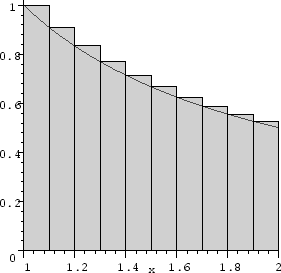The following is the result of using the Simpson's rule for the function xsin(x2) on the interval 1x5. Since the number of intervals is not specified, Maple assumes four equal intervals by default:

>
simpson(x*sin(x^2),x=1..5);## Multiple integrals

Maple does iterated integrals:
>
int(int(x^2*y^3,x=0..y),y=2..3);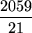This is literally an iterated integral: the integral of x2y3 with respect to x on the interval [0, y] is nested inside the integral with respect to y on the interval [2, 3], in effect calculating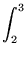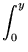x2y3dxdy .Next: The subs command Up: Preliminaries about Maple Previous: The diff (and Diff)

Translated from LaTeX by Scott Sutherland
2002-08-29